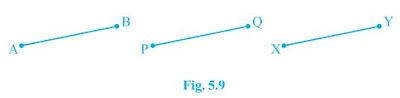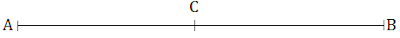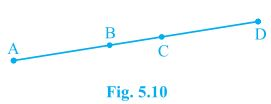>

# NCERT Solutions for Class 9 Maths Chapter 5 Introduction to Euclid’s Geometry| PDF Download

You will find Chapter 5 Introduction to Euclid’s Geometry Class 9 Maths NCERT Solutions on this page which are useful to understand the topics given in the chapter. Also, Class 9 Maths Chapter 5 Introduction to Euclid's Geometry PDF Download is available here through which one can study at your own pace. Chapter 5 Class 9 Maths NCERT Solutions will develop your skills and improving marks in the examinations.

Through the help of NCERT Solutions for Class 9 Maths, a student will be able to solve the difficult problems in a given in a exercise. These NCERT Solutions will help an individual to increase concentration and grasping key concepts of the chapter properly.Page No:85

Exercise 5.1

1. Which of the following statements are true and which are false? Give reasons for your
(i) Only one line can pass through a single point.
(ii) There are an infinite number of lines which pass through two distinct points.
(iii) A terminated line can be produced indefinitely on both the sides.
(iv) If two circles are equal, then their radii are equal.
(v) In Fig. 5.9, if AB = PQ and PQ = XY, then AB = XY.(i) False. There can be infinite line drawn passing through a single point.

(ii) False. Only one line can be drawn which passes through two distinct points.

(iii) True. A terminated line can be produced indefinitely on both the sides.
In geometry, a line can be extended in both direction. A line means infinite long length.

(iv) True. If two circles are equal, then their radii are equal.
By superposition, we will find that the centre and circumference of the both circles coincide. Hence, their radius must be equal.

(v) True. By Euclid's first axiom things which are equal to the same thing, are equal to one another.

2. Give a definition for each of the following terms. Are there other terms that need to be defined first? What are they, and how might you define them?
(i) parallel lines                 (ii) perpendicular lines                (iii) line segment
(iv) radius of a circle         (v) square

Yes, other terms need to be defined first which are:
Plane: A plane is flat surface on which geometric figures are drawn.
Point: A point is a dot drawn on a plane surface and is dimensionless.
Line: A line is collection of points which can extends in both direction and has only length not breadth.

(i) Parallel lines: When two or more never intersect each other in a plane and perpendicular distance between them is always constant then they are said to be parallel lines.

(ii) Perpendicular lines: When two lines intersect each other at right angle in a plane then they are said to be perpendicular to each other.

(iii) Line segment: A line segment is a part of a line with two end points and cannot be extended further.

(iv) Radius of circle: The fixed distance between the centre and the circumference of the circle is called the radius of the circle.

(v) Square: A square is a quadrilateral in which all the four sides are equal and each internal angle is a right angle.

3. Consider two ‘postulates’ given below:
(i) Given any two distinct points A and B, there exists a third point C which is in between A and B.
(ii) There exist at least three points that are not on the same line.
Do these postulates contain any undefined terms? Are these postulates consistent? Do they follow from Euclid’s postulates? Explain.

Undefined terms in the postulates:
→ Many points lie in a plane. But here it is not given about the position of the point C whether it lies on the line segment joining AB or not.
→ Also, there is no information about the plane whether the points are in same plane or not.
Yes, these postulates are consistent when we deal with these two situation:
(i) Point C is lying in between and on the line segment joining A and B.
(ii) Point C not lies on the line segment joining A and B.

Page No: 86

4. If a point C lies between two points A and B such that AC = BC, then prove that AC = 1/2 AB. Explain by drawing the figure.Here, AC = BC
AC + AC = BC + AC
also, BC +AC  = AB (as it coincides with line segment AB)
∴ 2 AC = AB (If equals are added to equals, the wholes are equal.)
⇒ AC = 1/2 AB.

5. In Question 4, point C is called a mid-point of line segment AB. Prove that every line segment has one and only one mid-point.Let A and B be the line segment and points P and Q be two different mid points of AB.
Now,
∴ P and Q are midpoints of AB.
Therefore AP=PB and also AQ = QB.
also, PB + AP = AB (as it coincides with line segment AB)
Similarly, QB + AQ = AB.
Now,
AP + AP = PB + AP (If equals are added to equals, the wholes are equal.)
⇒ 2 AP = AB --- (i)
Similarly,
2 AQ = AB --- (ii)
From (i) and (ii)
2 AP  = 2 AQ (Things which are equal to the same thing are equal to one another.)
⇒ AP = AQ (Things which are double of the same things are equal to one another.)
Thus, P and Q are the same points. This contradicts the fact that P and Q are two different mid points of AB. Thus, it is proved hat every line segment has one and only one mid-point.

6. In Fig. 5.10, if AC = BD, then prove that AB = CD.Given, AC = BD
From the figure,
AC = AB + BC
BD = BC + CD
⇒ AB + BC = BC + CD
According to Euclid's axiom, when equals are subtracted from equals, remainders are also equal.
Subtracting BC both sides,
AB + BC - BC = BC + CD - BC
AB = CD

7. Why is Axiom 5, in the list of Euclid’s axioms, considered a ‘universal truth’? (Note that the question is not about the fifth postulate.)

Axiom 5 : The whole is always greater than the part.
Take an example of a cake. When it is whole it will measures 2 pound but when we took out a part from it and measures its weigh it will came out lower than the previous one. So, the fifth axiom of Euclid is true for all the universal things. That is why it is considered a ‘universal truth’.

Page No: 88

Exercise 5.2

1. How would you rewrite Euclid’s fifth postulate so that it would be easier to understand?

The fifth postulates is about parallel lines.
When two or more never intersect each other in a plane and perpendicular distance between them is always constant then they are said to be parallel lines.
Two facts of the postulates:
(i) If P doesn't lie on l then we can draw a line through P which will be parallel to the line l.
(ii) There will be only one line can be drawn through P which is parallel to the line l.

2. Does Euclid’s fifth postulate imply the existence of parallel lines? Explain.

Yes, Euclid’s fifth postulate imply the existence of parallel lines.
If the sum of the interior angles will be equal to sum of the two right angles then two lines will not meet each other on either sides and therefore they will be parallel to each other.m and n will be parallel if
∠1 + ∠3 = 180°
Or  ∠3 + ∠4 = 180°

## NCERT Solutions for Class 9 Maths Chapter 5 Introduction to Euclid’s Geometry

Chapter 5 Introduction to Euclid’s Geometry is very helpful in knowing the concepts of geometry. Euclid was a Greek mathematician, who introduced the method of proving a geometrical result by using logical reasonings on previously proved and known results. In this chapter, we are going to learn various axioms and postulates of Euclid.

• Euclid’s Definitions, Axioms and Postulates: Some of the Euclid’s axioms are:
(i) The whole is greater than the part.
(ii) Things which are double of the same things are equal to one another.
(iii) Things which are halves of the same things are equal to one another.
(iv) Things which are equal to the same thing are equal to one another.
(v) Things which coincide with one another are equal to one another.
(vi) If equals are added to equals, the wholes are equal.
(vii ) If equals are subtracted from equals, the remainders are equal.

Euclid’s Five Postulates
Postulate 1: A straight line may be drawn from any point to any other point.
Postulate 2: A terminated line can be produced indefinitely.
Postulate 3: A circle can be drawn with any centre and any radius.
Postulate 4: All right angles are equal to one another.
Postulate 5: If a straight line falling on two straight lines makes the interior angles on the same side of it taken together is less than two right angles, then the two straight lines, if produced indefinitely, meet on that side on which the sum of the angles is less than two right angles.

• Equivalent Versions of Euclid’s Fifth Postulate: Euclid’s fifth postulate is very significant in the history of mathematics. It states that 'For every line l and for every point P not lying on l, there exists a unique line m passing through P and parallel to l' which can also be stated in the form 'Two distinct intersecting lines cannot be parallel to the same line.'

There are only two exercises in the Class 5 NCERT Solutions Class 9 Maths. We have also provided exercisewise NCERT Solutions which you can find below.

NCERT Solutions for Class 9 prepared by Studyrankers experts are very useful in clearing your doubts and enhancing your skills in a great way. By solving the questions from Class 9 NCERT textbook you will be able to solve supplementary books easily and efficiently.

### NCERT Solutions for Class 9 Maths Chapters:

 Chapter 1 Number Systems Chapter 2 Polynomials Chapter 3 Coordinate Geometry Chapter 4 Linear Equations in Two Variables Chapter 6 Lines and Angles Chapter 7 Triangles Chapter 8 Quadrilaterals Chapter 9 Areas of Parallelograms and Triangles Chapter 10 Circles Chapter 11 Constructions Chapter 12 Heron's Formula Chapter 13 Surface Areas And Volumes Chapter 14 Statistics Chapter 15 Probability

FAQ on Chapter 5 Introduction to Euclid’s Geometry

#### What are the benefits of NCERT Solutions for Chapter 5 Introduction to Euclid’s Geometry Class 9 NCERT Solutions?

Chapter 5 Introduction to Euclid’s Geometry Class  Maths NCERT Solutions is nice way through which one can make themselves doubt free and understand all the important points of the chapter. We have provided detailed NCERT Solutions of every questions that you can check here.

#### What is a theorem?

A mathematical statement whose truth has been logically established is called a theorem.

#### What is the Euclid’s second axiom?

If equals be added to equals, the wholes are equal.

#### How many dimensions does a surface has?

A surface has 2 dimensions.